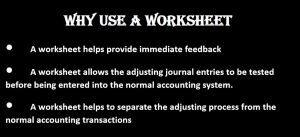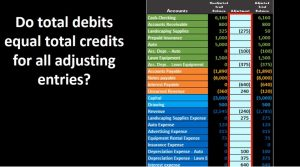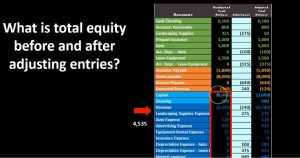## Why Use a Worksheet in Adjusting Process 3.5

Hello in this presentation we will discuss the reasoning for using a worksheet within the adjusting entry process, a worksheet like the one on the right where we have an unadjusted trial balance adjustments and then an adjusted trial balance. We typically think of this worksheet as outside of the normal journal entry process, meaning the normal journal entries that we are going to input will be in the general journal posted to the general ledger giving us more detail and then posted to the trial balance. And this case, we’re going to use a worksheet which will go straight to this adjusted column, and then show us the unadjusted balance the change and then the adjusted balance. If we were using accounting software like QuickBooks, then we would have the normal data input in the system we would produce then the unadjusted trial balance and put that into a worksheet such as this, and then work through this process to have our worksheet show the balances here. idea being that the worksheet in the adjusting process is going to be outside of the normal system.

01:05

When we think of the adjusting process, of course, we’re thinking of the process after the normal accounting journal entry. So normal checks, normal bills, normal invoices have been entered, then we have a separate, we can think of a separate department or even an outside CPA firm, who will be doing the adjusting process and therefore we will generate from our system a trial balance, which we will then within the adjusting process put into our worksheet and call the unadjusted trial balance, then make the adjustments and then have the adjusted trial balance finally ready for the use of the financial statements. So why use this worksheet? Why not just put it into the database system or it through the normal process, few benefits, a worksheets helps provide immediate feedback. So the worksheets going to give us the immediate input in terms of what’s going to happen to this account what’s going to happen to the two accounts effects What’s happened the net income.

02:01

And it gives it that that immediate feedback which we don’t see as easily when we have to post it to the general ledger and then to the trial balance. So when there’s only a few type of journal entries, it’s really helpful to have a little worksheet like this, that will give us a nice everything in one snapshot which we’ll see in a bit. A worksheet allows the adjusting journal entries to be tested before being entered into the normal accounting system. If we enter this this information into the adjusting process, the addition process is a little bit more complex because it does deal with the with the accrual entries, and therefore we do want to test it before we entered into the system and this worksheet can help us to provide that worksheet helps to separate the adjusting process from the normal accounting transactions. We do want to keep the worksheet or the adjusting process separate in our minds from the normal journal entry process so that we have the normal day to day journal entries.

02:56

And then we have these adjusting journal entries. If we keep them separate. It really helps To understand how the adjusting journal entries will work, and it helps us to basically have these two processes these two systems which kind of run on a on a different kind of spectrum that the accounting department does what it does, and then the adjusting entries do what they do. And it’s easier many times to have the accounting department not be interrupted by some of the adjustments in the adjusting department. So we’re going to, we’re going to think of them as a separate entity and or not a separate entity, we’re going to think of them as a separate process that we’ll be putting in place within the accounting system. And taking the information outside of the system and working it into a worksheet and then putting it back into the system helps us really define that separate process.

03:42

It also might be done by separate department or by an outside CPA firm, in which case we’ll also need to generate the trial balance from the system that will then be adjusted input back into the system. Some characteristics of the worksheet here once again, we have already counts, we’ve got the unadjusted trial balance, we have the adjustments, and then the adjusted trial balance. This will show us very quickly if we put this information into our trial balance, we can see very quickly that it is in balance. Now our system here is showing debits as positives, credits as negatives, rather than having two columns. For each of these. We had two columns for each, it would be six columns long, and a bit more complex to use Excel formulas. This brings it down to a really three columns, which is really nice to see and the formulas actually help.04:33

So in this case, we’re going to have positive numbers being debits negative numbers being credits, then if we were to say debits minus the credits, we end up with zero and we get that quick check to see that we are in balance in the unadjusted trial balance. We can also see that we are in balance in our adjustments. And it’s really nice that we only have a few adjustments that are going to happen typically we’re going to just basically five to 10 accounts possibly Because there’s only a few accounts, we can post those to the center column, rather than post them to the general ledger. And this allows us to see the effect very quickly. So we can see, for example, the landscaping supplies started here at the 325, changed by the 275 to the 50. At the end of the day, the reason we don’t do this type of method for all accounts is of course, if we had 10 things go into the landscaping supplies, then it would this into this journal entries column wouldn’t tell us much because there’d be too much information packed into this one cell. But if there’s only one account that’s being changed, if there’s only one journal entry, adjusting journal entry, changing this account, then it’s really nice to have a worksheet like this because it’ll give us a direct view of what is going on.

05:4506:55

And this is another way reason that if we have the revenue as credits and As the credits as negative numbers, which include revenue, and the debits as positive numbers, which include expenses, then we can really sum this stuff up and use Excel to give us a quick calculation of net income as well. So this will give us net income on the unadjusted trial balance very easily by just taking the credits, which are which are negative numbers here and then subtracting out the expenses. And that’s going to give us this net income. We do have to note however, if we’re using negative numbers for credits, that this doesn’t mean that we have a loss, it means that the credits are winning, because we have a credit balance that is over or greater than the debit balances, leaving us with that 2035 net income and the unadjusted trial balance. We can also see that net income in the adjusted trial balance of course, and we see it here. So here are our adjustments. We can see what the difference is, what did we do to these accounts, and then we can see The net income, once again very quickly calculated by Excel, we have the negative numbers, being credits positive being the expenses, and the debits and the credits of revenue minus the expenses of debits gives us net income, not a loss of 885.

08:18

In this case, we can also see what the activity is. So and this gives us a real show of what the impact of our adjustments are on the financial statements. So this is what our net income was before remember that income, not loss. If we add this column up, that won’t give us the change. So we of course have brought down net income by 1150. So we could see we could read it across this way we have the net income, and then we brought it down to 885. In this case, we can also group accounts really very quickly as well. We can take a look at the liabilities and within Excel, we can Just highlight these and Excel will sum them up, we don’t even have to use a calculator, we don’t have to use a sum function or anything, we can just highlight the cells. And Excel will give us that balance, in this case 10,250. We can do that, of course, for the adjusted trial balance as well, very easy for us to just sum this up, it’s actually a lot less bulky than looking at a balance sheet. And so we can test these accounts if we know what debits and credits are, this is a much cleaner way of seeing everything.

09:28

If we want to know what the total equity is, we can do that very quickly by just highlighting once again in Excel, just highlighting this range of cells and it will sum it up for us automatically the credits minus the debits here, so it’s going to take the credit minus the debit plus the credit minus the debit minus minus debit, giving us 4535. In this case, we can do the same for the adjusted trial balance. So this is different than net income down here because now we’re adding into this capital and draws and all we have to do is highlight this and it We’ll take the difference between the debits and the credits giving us a balance in this case of the total equity 3385. We can also see total equity and double check our accounting equation by adding up the assets minus the liabilities. And if we have this in the system as assets being positive numbers, because their debit balances and liabilities and credit numbers because they are credit balances, so negative numbers, then it will subtract this out for us.10:31

And we know that according to the accounting equation, assets minus liabilities will equal equity because assets equal liabilities plus equity. So in essence, if we take the assets minus the liability in the top half, it’ll, it’ll equal the credits minus the debits capital minus draws plus revenue minus expenses on the bottom half. And we can do that for the adjusted trial balance portion as well if we add up assets minus liabilities, we can just highlight those within Excel given us 3003 85. So the worksheet gives us a really quick view of all of this information, all the information we really need so that we can double check it, see what’s going on before we enter that into the system.